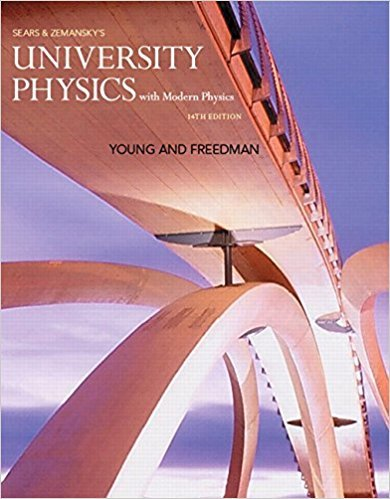×
Get Full Access to University Physics With Modern Physics (1) - 14 Edition - Chapter 17 - Problem 17.114
Get Full Access to University Physics With Modern Physics (1) - 14 Edition - Chapter 17 - Problem 17.114

×

# A Walk in the Sun. Consider a poor lost soul walking at 5ISBN: 9780321973610 228

## Solution for problem 17.114 Chapter 17

University Physics with Modern Physics (1) | 14th Edition

• Textbook Solutions
• 2901 Step-by-step solutions solved by professors and subject experts
• Get 24/7 help from StudySoup virtual teaching assistantsUniversity Physics with Modern Physics (1) | 14th Edition

4 5 1 361 Reviews
12
0
Problem 17.114

A Walk in the Sun. Consider a poor lost soul walking at 5 km>h on a hot day in the desert, wearing only a bathing suit. This persons skin temperature tends to rise due to four mechanisms: (i) energy is generated by metabolic reactions in the body at a rate of 280 W, and almost all of this energy is converted to heat that flows to the skin; (ii) heat is delivered to the skin by convection from the outside air at a rate equal to kAskin1Tair - Tskin2, where k is 54 J>h # C # m2 , the exposed skin area Askin is 1.5 m2 , the air temperature Tair is 47C, and the skin temperature Tskin is 36C; (iii) the skin absorbs radiant energy from the sun at a rate of 1400 W>m2 ; (iv) the skin absorbs radiant energy from the environment, which has temperature 47C. (a) Calculate the net rate (in watts) at which the persons skin is heated by all four of these mechanisms. Assume that the emissivity of the skin is e = 1 and that the skin temperature is initially 36C. Which mechanism is the most important? (b) At what rate 1in L>h2 must perspiration evaporate from this persons skin to maintain a constant skin temperature? (The heat of vaporization of water at 36C is 2.42 * 106 J>kg.) (c) Suppose instead the person is protected by light-colored clothing 1e 02 so that the exposed skin area is only 0.45 m2 . What rate of perspiration is required now? Discuss the usefulness of the traditional clothing worn by desert peoples.

Step-by-Step Solution:
Step 1 of 3

Lab 2: Kinematics Members: Samantha Rubin, Marguerite Furlong, Zoe Gaber Table Number: 2 Introduction Kinematics​ explores the relationship between objects and motion, particularly for this lab, an object in free fall. Velocity and acceleration are mathematically represented with their derivatives in respect to either position or velocity in terms of time: Here, Δx is identified as the initial position of x subtracted from the final position of x, and Δv, is the initial velocity subtracted from the final velocity. When acceleration is constant with respect to time, we can relate the following kinematic equations to define the relationships between position, velocity, and acceleration: Here, V r0fers to the initial velocity and X 0efers to the initial position, both in terms of time. The known acceleration is that of gravity, or the gravitational pull of the earth, which is defined as 9.8m/s . In this lab we examine the quantity of g ​​in the real life data collected from an object in free fall. Experiment 1 ­ Measuring the Acceleration by Gravity In this experiment a plastic rectangle with alternating clear and black horizontal stripes was dropped straight down, passing through a Photogate sensor. The plastic rectangle, referred to as a picket fence, measured 39cm and each black or clea

Step 2 of 3

Step 3 of 3

## Discover and learn what students are asking

Calculus: Early Transcendental Functions : Change of Variables: Polar Coordinates
?Describing a Region In Exercises 5-8, use polar coordinates to describe the region shown.

Statistics: Informed Decisions Using Data : Testing the Significance of the Least-Squares Regression Model
?Why don’t we conduct inference on the linear correlation coefficient?

Chemistry: The Central Science : Chemical Reactions and Reaction Stoichiometry
?A compound whose empirical formula is $$\mathrm{XF}_{3}$$ consists of 65 % F by mass. What is the atomic mass of X ?

Unlock Textbook Solution